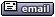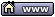flat assembler
Message board for the users of flat assembler.flat assembler > Examples and Tutorials > Hobby Basic, a toy language interpreter written in fasm Goto page 1, 2, 3  Next
Author
 ThreadPicnic

Joined: 05 May 2007
Posts: 1277
Location: countrysideHobby Basic is a toy language interpreter for the Windows console.
Its syntax resembles BASIC.
It's written in fasm + Win32 API.
It's a small personal project.

Last edited by Picnic on 16 Aug 2019, 09:21; edited 142 times in total05 May 2013, 21:16AsmGuru62

Joined: 28 Jan 2004
Posts: 1393
Great stuff!
Things like that motivate beginners to start programming.05 May 2013, 22:15MHajduk

Joined: 30 Mar 2006
Posts: 6023
Location: Poland
I suppose that the author's sentiment to QuickBasic was the main inspiration for this project. Nice thing.05 May 2013, 22:28typedef

Joined: 25 Jul 2010
Posts: 2909
Location: 0x77760000
Nice. This prompts me to make a CLI graphics library. Looks pretty neat.07 May 2013, 04:31Picnic

Joined: 05 May 2007
Posts: 1277
Location: countryside
I am glad you like it, i had some concern whether it can be served as helpful example despite the lack of comments in source.

A test program for the interpreter (80x25 screen).
Code:
```
rem  2D rally car

LEFT=37
RIGHT=39

dim rally[9,5]
a\$="███                     ███"

path\$=PATH(1)+"\ART\RALLY"
file path\$,rally[],1

path\$=PATH(1)+"\DATA\rally.txt"
if SIZE(path\$)=-1 then file path\$,'100',0

cursor 0,0
screen 80,25
grab 0,0,9,5,rally[],1
copy 0,0,9,5

start#

x=36
y=12
n=30
sc=0
t=40000

file path\$,best\$,1
best\$=TRIM(best\$)

color 0,7:cls
at 4,2,"Use the arrow keys"
at 4,3,("Try to beat "+best\$)
at 4,4,"Distance "
color 6,2

for i=0 to 24
erase n,i,24,-1,1
at n,i,a\$
next

main#

paint x,y-1,9,5,-1,-1

if KEY(LEFT)
x=x-1
elseif KEY(RIGHT)
x=x+1
elseif KEY(27)
end
endif

if (CELL(x,y+2)='█' or CELL(x+1,y+4)='█' or CELL(x+8,y+2)='█' or CELL(x+7,y+4)='█')
paste x,y
flip x,y,9,5,1
invert x,y,9,5
if sc>VAL(best\$)
best\$=STR(sc)
file path\$,best\$,0
endif
wait 1000000
goto start
endif

n=n+rnd(3)-1
if n<25 then n=25
if n>48 then n=48

paste x,y
color 0,0
move 25,1,50,24,25,0
color 6,2
erase n,24,25,-1,1
at n,24,a\$

sc=sc+1
pen 7:at 13,4,sc

if t>15000 then t=t-20
wait t

goto main
```Last edited by Picnic on 16 Aug 2019, 11:17; edited 6 times in total07 May 2013, 10:10TmX

Joined: 02 Mar 2006
Posts: 820
Location: Jakarta, Indonesia
This is very nice.
Reminiscent of DOS apps.

Mouse handling would be a nice addition, I think07 May 2013, 15:45HaHaAnonymous

Joined: 02 Dec 2012
Posts: 1181
Location: Unknown
Stupid post removed.

Last edited by HaHaAnonymous on 28 Feb 2015, 20:54; edited 1 time in total07 May 2013, 20:58typedef

Joined: 25 Jul 2010
Posts: 2909
Location: 0x77760000
Picnic wrote:
I am glad you like it, i had some concern whether it can be served as helpful example despite the lack of comments in source.

If it can be understood then no worries.

Besides not all examples are aimed at beginners.07 May 2013, 23:30Picnic

Joined: 05 May 2007
Posts: 1277
Location: countryside
TmX wrote:
Mouse handling would be a nice addition, I thinkMouse events handling added in Hobby Basic.Code:
```
do

mouse

! V0 = cell x
! V1 = cell y
! V2 = button: 1 left, 2 right, 4 double click
! V3 = coordinates in pixels (high/low order word)

title V0,',',V1

wait 1

until KEY(27)
```Last edited by Picnic on 16 Aug 2019, 09:20; edited 11 times in total18 Aug 2013, 22:52Picnic

Joined: 05 May 2007
Posts: 1277
Location: countryside
A new version, the first that supports unicode characters in 16-bit encoding.

Code:
```
cls
τύπωσε  fix  print
τύπωσε "Euro Symbol: €."
τύπωσε "English: I can eat glass and it doesn''t hurt me."
τύπωσε "Greek: Μπορώ να φάω γυαλί χωρίς να πάθω τίποτα."
τύπωσε "Íslenska / Icelandic: Ég get etið gler án þess að meiða mig."
τύπωσε "Romanian: Pot să mănânc sticlă și ea nu mă rănește."
τύπωσε "Ukrainian: Я можу їсти шкло, й воно мені не пошкодить."
τύπωσε "Polish: Mogę jeść szkło, i mi nie szkodzi."
τύπωσε "Czech: Mohu jíst sklo, neublíží mi."
pause
```Last edited by Picnic on 16 Aug 2019, 11:17; edited 7 times in total15 Dec 2013, 01:37typedef

Joined: 25 Jul 2010
Posts: 2909
Location: 0x77760000
I get this when I run MOUSE15 Dec 2013, 05:58Picnic

Joined: 05 May 2007
Posts: 1277
Location: countryside
Thanks for the feedback typedef. Can you please tell me your Windows version and if you tried the rest of the samples?15 Dec 2013, 10:10typedef

Joined: 25 Jul 2010
Posts: 2909
Location: 0x77760000
Windows 7 64 bit. Actually all the programs except the binary and the the color ones. And why it says print I don't know15 Dec 2013, 14:27Picnic

Joined: 05 May 2007
Posts: 1277
Location: countryside
I will look into the problem as soon as i can. I starting to feel a bit outdated with my windows xp 32-bit.

Last edited by Picnic on 15 Dec 2013, 17:35; edited 1 time in total15 Dec 2013, 17:28typedef

Joined: 25 Jul 2010
Posts: 2909
Location: 0x77760000
I see. It's a version difference thing.15 Dec 2013, 17:34Picnic

Joined: 05 May 2007
Posts: 1277
Location: countryside
I think i found what was causing the trouble. Give it a try if you like typedef.18 Dec 2013, 21:33typedef

Joined: 25 Jul 2010
Posts: 2909
Location: 0x77760000
They work now. Care to share what was causing it to say that?18 Dec 2013, 22:53Picnic

Joined: 05 May 2007
Posts: 1277
Location: countryside
Nice to hear!
Sure, it seems that an improper call to SetConsoleOutputCP function crush the program. By the way, i installed Windows 7.19 Dec 2013, 21:09typedef

Joined: 25 Jul 2010
Posts: 2909
Location: 0x77760000
Picnic wrote:
Nice to hear!
Sure, it seems that an improper call to SetConsoleOutputCP function crush the program. By the way, i installed Windows 7.

Hmm. Nice, what Win7 version?20 Dec 2013, 01:13Picnic

Joined: 05 May 2007
Posts: 1277
Location: countryside
A new version upload with some minor improvements, and fixes in bugs i noticed.

X's And O's (Tic Tac Toe) game. Plays with mouse.
Code:
```
rem  X's and O's Game

P1  fix  m[x,y]
P2  fix  m[x+i,y+j]
P3  fix  m[x+i*2,y+j*2]

screen 80,30
screen 80,30
cursor 0,0

restart#
color 0,7:cls:locate 0,15
n=0:dim m[3,3]=0,0,0,0,0,0,0,0,0
at 4,1,"X''s and O''s"
at 0,3,"Player X  vs  CPU O"
paint 1,7,17,1,'_',0
paint 1,10,17,1,'_',0
paint 6,5,1,9,'|',0
paint 12,5,1,9,'|',0
@@# at 0,15,"Play first? [y/n]"
inkey:p=V0:if p=27 then end
if (p='y' or p='Y') then p=1 else p=0
at 0,15,"Strong CPU? [y/n]"
inkey:z=V0:if z=27 then goto @b
do
if p=0 then gosub cpu_move else gosub player_move
gosub is_winner:p=p^1:n=n+1
until (w<>0 or n=9)
erase 0,15,30,-1,-1:pen 7
if w=0 then at 0,15,"Game was a draw"
if w=1 then at 0,15,"Player X has won!"
if w=2 then at 0,15,"CPU O has won!"
at 0,16,"press a key":inkey:goto restart

player_move#
pen 7:at 0,15,"Player X''s turn       "
@@# if key(27) then goto restart
mouse:x=(V0+1)/7:y=(V1+1)/4-1:btn=V2:wait 1
if (x<0 or x>2 or y<0 or y>2) or btn=0 then goto @b
if m[x,y]=0 then m[x,y]=1:pen 10:at x*6+3,y*3+6,'X':return
goto @b

cpu_move#
pen 7:at 0,15,"CPU O''s turn          "
b=0:cx=0:cy=0
x=0:i=1:j=0:for y=0 to 2:gosub scoring:next
y=0:i=0:j=1:for x=0 to 2:gosub scoring:next
x=2:y=0:i=-1:j=1:gosub scoring
x=0:i=1:j=1:gosub scoring
m[cx,cy]=2:wait 500000
pen 15:at cx*6+3,cy*3+6,'O'
return

scoring#
r=0:k=0:s=0
if P1=0 then r=x:k=y:s=1
if P2=0 then r=x+i:k=y+j:s=1
if P3=0 then r=x+i*2:k=y+j*2:s=1
if (P1=1 and P2=0 and P3=0) then r=x+i*2:k=y+j*2:s=2
if (P1=0 and P2=1 and P3=0) then r=x:k=y:s=2
if (P1=0 and P2=0 and P3=1) then r=x:k=y:s=2
if (P1=0 and P2=0 and P3=0) then r=x:k=y:s=4
if (P1=2 and P2=0 and P3=0) then r=x+i*2:k=y+j*2:s=8
if (P1=0 and P2=2 and P3=0) then r=x:k=y:s=8
if (P1=0 and P2=0 and P3=2) then r=x:k=y:s=8
if (P1=1 and P2=1 and P3=0) then r=x+i*2:k=y+j*2:s=16
if (P1=1 and P2=0 and P3=1) then r=x+i:k=y+j:s=16
if (P1=0 and P2=1 and P3=1) then r=x:k=y:s=16
if (P1=2 and P2=2 and P3=0) then r=x+i*2:k=y+j*2:s=32
if (P1=2 and P2=0 and P3=2) then r=x+i:k=y+j:s=32
if (P1=0 and P2=2 and P3=2) then r=x:k=y:s=32
if (m[1,1]=0 and z='y') then r=1:k=1:s=64
if s>b then b=s:cx=r:cy=k
return

is_winner#
w=0:x=0:i=1:j=0:for y=0 to 2:gosub win:next
y=0:i=0:j=1:for x=0 to 2:gosub win:next
x=2:y=0:i=-1:j=1:gosub win
x=0:i=1:j=1:gosub win
return

win#
if (P1=1 and P2=1 and P3=1) then w=1
if (P1=2 and P2=2 and P3=2) then w=2
return
```Last edited by Picnic on 16 Aug 2019, 11:18; edited 4 times in total20 Dec 2013, 20:37Display posts from previous: All Posts1 Day7 Days2 Weeks1 Month3 Months6 Months1 Year Oldest FirstNewest FirstJump to: Select a forum Official----------------AssemblyPeripheria General----------------MainDOSWindowsLinuxUnixMenuetOS Specific----------------MacroinstructionsCompiler InternalsIDE DevelopmentOS ConstructionNon-x86 architecturesHigh Level LanguagesProgramming Language DesignProjects and IdeasExamples and Tutorials Other----------------FeedbackHeapTest Area
Goto page 1, 2, 3  Next

Forum Rules:
 You cannot post new topics in this forumYou cannot reply to topics in this forumYou cannot edit your posts in this forumYou cannot delete your posts in this forumYou cannot vote in polls in this forumYou cannot attach files in this forumYou can download files in this forum